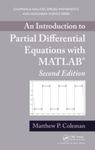## Mathematics Faculty Book Gallery#### Title

An Introduction to Partial Differential Equations with MATLAB, 2nd edition

Book

#### Description/Summary

An Introduction to Partial Differential Equations with MATLAB®, Second Edition illustrates the usefulness of PDEs through numerous applications and helps students appreciate the beauty of the underlying mathematics. Updated throughout, this second edition of a bestseller shows students how PDEs can model diverse problems, including the flow of heat, the propagation of sound waves, the spread of algae along the ocean’s surface, the fluctuation in the price of a stock option, and the quantum mechanical behavior of a hydrogen atom.

Suitable for a two-semester introduction to PDEs and Fourier series for mathematics, physics, and engineering students, the text teaches the equations based on method of solution. It provides both physical and mathematical motivation as much as possible. The author treats problems in one spatial dimension before dealing with those in higher dimensions. He covers PDEs on bounded domains and then on unbounded domains, introducing students to Fourier series early on in the text.

Each chapter’s prelude explains what and why material is to be covered and considers the material in a historical setting. The text also contains many exercises, including standard ones and graphical problems using MATLAB. While the book can be used without MATLAB, instructors and students are encouraged to take advantage of MATLAB’s excellent graphics capabilities. The MATLAB code used to generate the tables and figures is available in an appendix and on the author’s website. -- Publisher description.

9781439898468

2013

#### Publication Information

Coleman, Matthew P. An introduction to partial differential equations with MATLAB. CRC Press, 2013.